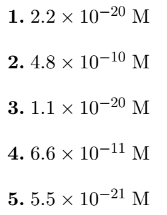# Problem: What is the minimum S2− concentration that will cause ZnS to start precipitating from a 0.10 M solution of Zn(NO3)2, a very soluble salt? Ksp for ZnS is 1.1 × 10−21.

###### FREE Expert Solution
95% (264 ratings)
###### FREE Expert Solution

For this problem, we’re being asked to calculate the concentration of S2– needed in order to precipitate ZnS from a 0.10 M Zn(NO3)2 solution.

Since the compounds are ionic compounds, they form ions when dissociating in water. The dissociation of ZnS and Zn(NO3)2 in water are as follows:

The sulfide ion, S2–, has a charge of –2. Zinc then has a charge of +2:

ZnS(s)  Zn2+(aq) + S2–(aq)

The nitrate ion, NO3, has a charge of –1. Zinc then has a charge of +2:

Zn(NO3)2  Zn2+(aq) + 2 NO3(aq)

95% (264 ratings)###### Problem Details

What is the minimum S2− concentration that will cause ZnS to start precipitating from a 0.10 M solution of Zn(NO3)2, a very soluble salt? Ksp for ZnS is 1.1 × 10−21.What scientific concept do you need to know in order to solve this problem?

Our tutors have indicated that to solve this problem you will need to apply the Ksp concept. You can view video lessons to learn Ksp. Or if you need more Ksp practice, you can also practice Ksp practice problems.

What is the difficulty of this problem?

Our tutors rated the difficulty ofWhat is the minimum S2− concentration that will cause ZnS to...as medium difficulty.

How long does this problem take to solve?

Our expert Chemistry tutor, Jules took 3 minutes and 17 seconds to solve this problem. You can follow their steps in the video explanation above.

What professor is this problem relevant for?

Based on our data, we think this problem is relevant for Professor Laude's class at TEXAS.